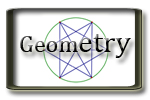G.P. Geometry Unit 11 Volume Jump to Unit 1 - 2 - 3 - 4 - 5 - 6 - 7- 8- 9 - 10 - 11 Big Ideas Video Tutorials - Lots of video examples for Units 8 -11

# Unit 11

Lesson

Handouts/Activities

Videos and Support Resources

11.1 Circumference and Arc Length
 Example 1 Example 2 Example 3 Example 4 Example 5
11.2 Areas of Circles and Sectors
 Example 1 Example 2 Example 3 Example 4 Example 5
11.3 Areas of Polygons
 Example 1 Example 2 Example 3 Example 4
11.4 3-Dimensional Figures
 Example 1 Example 2 Example 3
11.5 Volumes of Prisms and Cylinders
 Example 1 Example 2 Example 3 Example 4 Example 5 Example 6 Example 7
11.6 Volumes of Pyramids
• Notes
• Assignment
 Example 1 Example 2 Example 3 Example 4 Example 5
11.7 Surface Areas and Volumes of Spheres
• Notes
• Assignment
 Example 1 Example 2 Example 3 Example 4
11.8 Surface Area and volumes of Spheres
• Notes
• Assignment
 Example 1 Example 2 Example 3 Example 4 Example 5